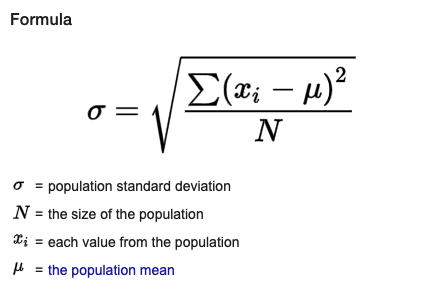# tools

Calculate Standard Deviation | Standard Deviation Online | Math Tools

# Calculate Standard Deviation Online Tool

### About Standard Deviation Calculator ToolThis is a free online tool to do calculation of standard deviation statistics function.

### Why Standard Deviation Calculation Is Needed?

Standard deviation is a common way to measure variation or dispersion of data. This is a common tool to do statistical analysis of large data set.

### What Standard Deviation Formula is used this tool?

This tool uses mean to calculate the variation.### What separator to use in the data in this tool?

This tool tries to automatically guess from the list of common separators that include following. In case data has multiple separator it will use following order

`Newline: '\n'`
`Comma: ','`
`Colon: ':'`
`Semicolon: ';'`
`Tab: '\t'`
`PIpe: '|'`